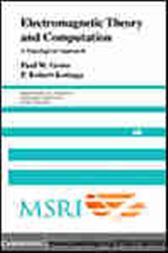Electromagnetic Theory and Computation

Series: Mathematical Sciences Research Institute Publications (No. 48)CA\$54.05
ISBNs
• 9780521801607
• 9780511207945
Although topology was recognized by Gauss and Maxwell to play a pivotal role in the formulation of electromagnetic boundary value problems, it is a largely unexploited tool for field computation. The development of algebraic topology since Maxwell provides a framework for linking data structures, algorithms, and computation to topological aspects of three-dimensional electromagnetic boundary value problems. This book attempts to expose the link between Maxwell and a modern approach to algorithms. The first chapters lay out the relevant facts about homology and cohomology, stressing their interpretations in electromagnetism. These topological structures are subsequently tied to variational formulations in electromagnetics, the finite element method, algorithms, and certain aspects of numerical linear algebra. A recurring theme is the formulation of and algorithms for the problem of making branch cuts for computing magnetic scalar potentials and eddy currents.

ISBNs
• 9780521801607
• 9780511207945